Courses

# Free Energy and its Connection with Thermodynamic Quantities - CSIR-NET Physical Sciences Physics Notes | EduRev

## Physics : Free Energy and its Connection with Thermodynamic Quantities - CSIR-NET Physical Sciences Physics Notes | EduRev

The document Free Energy and its Connection with Thermodynamic Quantities - CSIR-NET Physical Sciences Physics Notes | EduRev is a part of the Physics Course Physics for IIT JAM, UGC - NET, CSIR NET.
All you need of Physics at this link: Physics

The thermodynamic free energy is the amount of work that a thermodynamic system can perform. The concept is useful in the thermodynamics of chemical or thermal processes in engineering and science. The free energy is the internal energy of a system minus the amount of energy that cannot be used to perform work. This unusable energy is given by the entropy of a system multiplied by the temperature of the system.

Like the internal energy, the free energy is a thermodynamic state function. Energy is a generalization of free energy, since energy is the ability to do work which is free energy.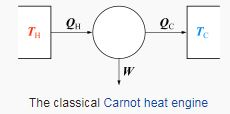Overview

Free energy is that portion of any first-law energy that is available to perform thermodynamic work; i.e., work mediated by thermal energy. Free energy is subject to irreversible loss in the course of such work. Since first-law energy is always conserved, it is evident that free energy is an expendable, second-law kind of energy that can perform work within finite amounts of time. Several free energy functions may be formulated based on system criteria. Free energy functions are Legendre transformations of the internal energy. For processes involving a system at constantpressure p and temperature T, the Gibbs free energy is the most useful because, in addition to subsuming any entropy change due merely to heat, it does the same for the p dV work needed to "make space for additional molecules" produced by various processes. (Hence its utility to solution-phase chemists, including biochemists.) The Helmholtz free energy has a special theoretical importance since it is proportional to the logarithm of the partition function for the canonical ensemble in statistical mechanics. (Hence its utility to physicists; and to gas-phase chemists and engineers, who do not want to ignore p dV work.)

The historically earlier Helmholtz free energy is defined as A = U − TS, where U is the internal energy, T is the absolute temperature, and S is the entropy. Its change is equal to the amount of reversible work done on, or obtainable from, a system at constant T. Thus its appellation "work content", and the designation A from Arbeit, the German word for work. Since it makes no reference to any quantities involved in work (such as p and V), the Helmholtz function is completely general: its decrease is the maximum amount of work which can be done by a system, and it can increase at most by the amount of work done on a system.

The Gibbs free energy is given by G = H − TS, where H is the enthalpy. (H = U + pV, where p is the pressure and V is the volume.)

These energy terms have been used inconsistently. In physics, free energy most often refers to the Helmholtz free energy, denoted by A, while in chemistry, free energymost often refers to the Gibbs free energy. Since both fields use both functions, a compromise has been suggested, using A to denote the Helmholtz function and G for the Gibbs function. While A is preferred by IUPAC, G is sometimes still in use, and the correct free energy function is often implicit in manuscripts and presentations.

Meaning of "free"

The basic definition of "energy" is a measure of a body's (in thermodynamics, the system) ability to cause change. For example, when a person pushes a heavy box a few meters forward, that person utilizes energy by exerting it in the form of mechanical energy, also known as work, on the box by a distance of a few meters forward. The mathematical definition of this form of energy is the product of the force exerted on the object and the distance by which the box moved (Work=Force x Distance). Because the person changed the stationary position of the box, that person exerted energy on that box. The work exerted is also called "useful energy" because all of the energy from the person went into moving the box. Because energy is neither created nor destroyed, but conserved (1st Law of Thermodynamics), it is constantly being converted from one form into another. For the case of the person pushing the box, the energy in the form of internal (or potential) energy obtained through metabolism was converted into work in order to push the box. This energy conversion, however, is not linear. In other words, some internal energy went into pushing the box, whereas some was lost in the form of heat (thermal energy). The difference of the internal energy, which is defined by U and the energy lost while performing work, usually in the form of heat, which can be defined as the product of the absolute temperature T and the entropy S (entropy is a measure of disorder in a system; more specifically, the measure of the thermal energy not available to perform work) of a body is what is called the "useful energy" of the body, or the work of the body performed on an object. In thermodynamics, this is what is known as "free energy". In other words, free energy is a measure of work (useful energy) a system can perform. Mathematically, free energy is expressed as:

free energy= U-TS

This expression means that free energy (energy of a system available to perform work) is the difference of the total internal energy of a system, and the energy not available to perform work, altered by the absolute temperature of the system, also known as entropy.

In the 18th and 19th centuries, the theory of heat, i.e., that heat is a form of energy having relation to vibratory motion, was beginning to supplant both the caloric theory, i.e., that heat is a fluid, and the four element theory, in which heat was the lightest of the four elements. In a similar manner, during these years, heat was beginning to be distinguished into different classification categories, such as “free heat”, “combined heat”, “radiant heat”, specific heat, heat capacity, “absolute heat”, “latent caloric”, “free” or “perceptible” caloric (calorique sensible), among others.

In 1780, for example, Laplace and Lavoisier stated: “In general, one can change the first hypothesis into the second by changing the words ‘free heat, combined heat, and heat released’ into ‘vis viva, loss of vis viva, and increase of vis viva.’” In this manner, the total mass of caloric in a body, called absolute heat, was regarded as a mixture of two components; the free or perceptible caloric could affect a thermometer, whereas the other component, the latent caloric, could not. The use of the words “latent heat” implied a similarity to latent heat in the more usual sense; it was regarded as chemically bound to the molecules of the body. In the adiabatic compression of a gas, the absolute heat remained constant but the observed rise in temperature implied that some latent caloric had become “free” or perceptible.

During the early 19th century, the concept of perceptible or free caloric began to be referred to as “free heat” or heat set free. In 1824, for example, the French physicist Sadi Carnot, in his famous “Reflections on the Motive Power of Fire”, speaks of quantities of heat ‘absorbed or set free’ in different transformations. In 1882, the German physicist and physiologist Hermann von Helmholtz coined the phrase ‘free energy’ for the expression E − TS, in which the change in F (or G) determines the amount of energy ‘free’ for work under the given conditions.

Thus, in traditional use, the term “free” was attached to Gibbs free energy, i.e., for systems at constant pressure and temperature, or to Helmholtz free energy, i.e., for systems at constant volume and temperature, to mean ‘available in the form of useful work.’ With reference to the Gibbs free energy, we add the qualification that it is the energy free for non-volume work.

An increasing number of books and journal articles do not include the attachment “free”, referring to G as simply Gibbs energy (and likewise for the Helmholtz energy). This is the result of a 1988 IUPAC meeting to set unified terminologies for the international scientific community, in which the adjective ‘free’ was supposedly banished. This standard, however, has not yet been universally adopted, and many published articles and books still include the descriptive ‘free’.[citation needed]

Application

Just like with the general concept of energy, free energy has multiple definitions, depending on the conditions. In physics, chemistry, and biology, these conditions are thermodynamic parameters (Temperature T, Volume V, Pressure P, etc.). Scientists have come up with many ways to define free energy while keeping certain parameters from changing; mathematically expressed as (. When temperature and volume are kept constant, this is known as Helmholtz free energy A. The mathematical expression of Helmholtz free energy is: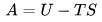This definition of free energy is useful in physics for explaining the behavior of isolated systems kept at a constant volume. In chemistry, on the other hand, most chemical reactions are kept at constant pressure. Under this condition, the heat of the reaction q is equal to the enthalpy H of the reaction, or all of the energy associated with the reaction. For example, if a researcher wanted to perform a combustion reaction in a bomb calorimeter, the pressure is kept constant throughout the course of a reaction per mole of the substance of the system (reactants and products). Therefore, the heat of the reaction is a direct measure of the enthalpy H of the reaction (q=). In this case, internal energy can be a measure of the enthalpy of the reaction in question (H=U). Thus under constant pressure and temperature, the free energy in a reaction is known as Gibbs free energy G.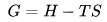The experimental usefulness of these functions is restricted to conditions where certain variables (T, and V or external p) are held constant, although they also have theoretical importance in deriving Maxwell relations. Work other than p dV may be added, e.g., for electrochemical cells, or f dx work in elastic materials and in muscle contraction. Other forms of work which must sometimes be considered are stress-strain, magnetic, as in adiabatic demagnetization used in the approach to absolute zero, and work due to electric polarization. These are described by tensors.

In most cases of interest there are internal degrees of freedom and processes, such as chemical reactions and phase transitions, which create entropy. Even for homogeneous "bulk" materials, the free energy functions depend on the (often suppressed) composition, as do all proper thermodynamic potentials (extensive functions), including the internal energy.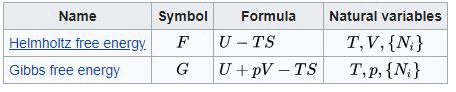Ni is the number of molecules (alternatively, moles) of type i in the system. If these quantities do not appear, it is impossible to describe compositional changes. The differentials for reversible processes are (assuming only pV work):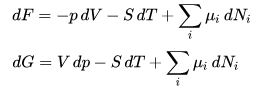where μi is the chemical potential for the ith component in the system. The second relation is especially useful at constant T and p, conditions which are easy to achieve experimentally, and which approximately characterize living creatures.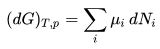Any decrease in the Gibbs function of a system is the upper limit for any isothermal, isobaric work that can be captured in the surroundings, or it may simply be dissipated, appearing as T times a corresponding increase in the entropy of the system and/or its surrounding.

An example is surface free energy, the amount of increase of free energy when the area of surface increases by every unit area.

The path integral Monte Carlo method is a numerical approach for determining the values of free energies, based on quantum dynamical principles.

History

The quantity called "free energy" is a more advanced and accurate replacement for the outdated term affinity, which was used by chemists in previous years to describe the forcethat caused chemical reactions. The term affinity, as used in chemical relation, dates back to at least the time of Albertus Magnus in 1250.

From the 1998 textbook Modern Thermodynamics by Nobel Laureate and chemistry professor Ilya Prigogine we find: "As motion was explained by the Newtonian concept of force, chemists wanted a similar concept of ‘driving force’ for chemical change. Why do chemical reactions occur, and why do they stop at certain points? Chemists called the ‘force’ that caused chemical reactions affinity, but it lacked a clear definition."

During the entire 18th century, the dominant view with regard to heat and light was that put forth by Isaac Newton, called the Newtonian hypothesis, which states that light and heat are forms of matter attracted or repelled by other forms of matter, with forces analogous to gravitation or to chemical affinity.

In the 19th century, the French chemist Marcellin Berthelot and the Danish chemist Julius Thomsen had attempted to quantify affinity using heats of reaction. In 1875, after quantifying the heats of reaction for a large number of compounds, Berthelot proposed the principle of maximum work, in which all chemical changes occurring without intervention of outside energy tend toward the production of bodies or of a system of bodies which liberate heat.

In addition to this, in 1780 Antoine Lavoisier and Pierre-Simon Laplace laid the foundations of thermochemistry by showing that the heat given out in a reaction is equal to the heat absorbed in the reverse reaction. They also investigated the specific heat and latent heat of a number of substances, and amounts of heat given out in combustion. In a similar manner, in 1840 Swiss chemist Germain Hess formulated the principle that the evolution of heat in a reaction is the same whether the process is accomplished in one-step process or in a number of stages. This is known as Hess' law. With the advent of the mechanical theory of heat in the early 19th century, Hess’s law came to be viewed as a consequence of the law of conservation of energy.

Based on these and other ideas, Berthelot and Thomsen, as well as others, considered the heat given out in the formation of a compound as a measure of the affinity, or the work done by the chemical forces. This view, however, was not entirely correct. In 1847, the English physicist James Joule showed that he could raise the temperature of water by turning a paddle wheel in it, thus showing that heat and mechanical work were equivalent or proportional to each other, i.e., approximately, dW ∝ dQ. This statement came to be known as the mechanical equivalent of heat and was a precursory form of the first law of thermodynamics.

By 1865, the German physicist Rudolf Clausius had shown that this equivalence principle needed amendment. That is, one can use the heat derived from a combustion reaction in a coal furnace to boil water, and use this heat to vaporize steam, and then use the enhanced high-pressure energy of the vaporized steam to push a piston. Thus, we might naively reason that one can entirely convert the initial combustion heat of the chemical reaction into the work of pushing the piston. Clausius showed, however, that we must take into account the work that the molecules of the working body, i.e., the water molecules in the cylinder, do on each other as they pass or transform from one step of or state of the engine cycle to the next, e.g., from (P1,V1) to (P2,V2). Clausius originally called this the “transformation content” of the body, and then later changed the name to entropy. Thus, the heat used to transform the working body of molecules from one state to the next cannot be used to do external work, e.g., to push the piston. Clausius defined this transformation heat as dQ = T dS.

In 1873, Willard Gibbs published A Method of Geometrical Representation of the Thermodynamic Properties of Substances by Means of Surfaces, in which he introduced the preliminary outline of the principles of his new equation able to predict or estimate the tendencies of various natural processes to ensue when bodies or systems are brought into contact. By studying the interactions of homogeneous substances in contact, i.e., bodies, being in composition part solid, part liquid, and part vapor, and by using a three-dimensional volume-entropy-internal energy graph, Gibbs was able to determine three states of equilibrium, i.e., "necessarily stable", "neutral", and "unstable", and whether or not changes will ensue. In 1876, Gibbs built on this framework by introducing the concept of chemical potential so to take into account chemical reactions and states of bodies that are chemically different from each other. In his own words, to summarize his results in 1873, Gibbs states:

 If we wish to express in a single equation the necessary and sufficient condition of thermodynamic equilibrium for a substance when surrounded by a medium of constant pressure p and temperature T, this equation may be written:δ(ε − Tη + pν) = 0when δ refers to the variation produced by any variations in the state of the parts of the body, and (when different parts of the body are in different states) in the proportion in which the body is divided between the different states. The condition of stable equilibrium is that the value of the expression in the parenthesis shall be a minimum.

In this description, as used by Gibbs, ε refers to the internal energy of the body, η refers to the entropy of the body, and ν is the volume of the body.

Hence, in 1882, after the introduction of these arguments by Clausius and Gibbs, the German scientist Hermann von Helmholtz stated, in opposition to Berthelot and Thomas’ hypothesis that chemical affinity is a measure of the heat of reaction of chemical reaction as based on the principle of maximal work, that affinity is not the heat given out in the formation of a compound but rather it is the largest quantity of work which can be gained when the reaction is carried out in a reversible manner, e.g., electrical work in a reversible cell. The maximum work is thus regarded as the diminution of the free, or available, energy of the system (Gibbs free energy G at T = constant, P = constant or Helmholtz free energyF at T = constant, V = constant), whilst the heat given out is usually a measure of the diminution of the total energy of the system (Internal energy). Thus, G or F is the amount of energy “free” for work under the given conditions.

Up until this point, the general view had been such that: “all chemical reactions drive the system to a state of equilibrium in which the affinities of the reactions vanish”. Over the next 60 years, the term affinity came to be replaced with the term free energy. According to chemistry historian Henry Leicester, the influential 1923 textbook Thermodynamics and the Free Energy of Chemical Reactions by Gilbert N. Lewis and Merle Randall led to the replacement of the term “affinity” by the term “free energy” in much of the English-speaking world.

159 docs

,

,

,

,

,

,

,

,

,

,

,

,

,

,

,

,

,

,

,

,

,

;